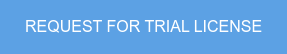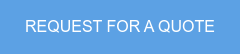Overview of the Training
1) Introduction of analysis procedure of completed state analysis and construction stage analysis
1-1) Completed state analysis (Linearized Finite Displacement)
1-2)Construction stage analysis (Backward; Geometric Non Linear)
2) Overview of suspension bridge project
3) Modeling suspension bridge in midas Civil

Introduction
The analysis of a suspension bridge is divided into completed state analysis and construction stage analysis. The completed state analysis is performed to check the behavior of the completed bridge. At this stage, the structure is in balance under self-weight and the deflection due to the self-weight has already occurred. This stage is referred to as the initial equilibrium state of the suspension bridge. The initial equilibrium state analysis will provide the coordinates and tension forces in the cables. The completed state analysis of the suspension bridge is performed to check the behavior of the structure under additional loads such as live, seismic and wind loadings. The selfweight loading in the initial equilibrium state will also be added to the total loading for the completed stage analysis.

Suspension bridges exhibit significant nonlinear behavior during the construction stages. But it can be assumed that the bridge behaves linearly for additional loads (vehicle, wind load, etc.) in the completed state analysis. This is due to the fact that sufficient tension forces are induced into the main cables and hangers under the initial equilibrium state loading. It is thus possible to perform a linearized analysis for the additional static loads at the completed state by converting the tension forces in the main cables and hangers resulting from the initial equilibrium state loading into increased geometric stiffness of those components. This linearized analytical procedure to convert section forces to geometric stiffness is referred to as the linearized finite displacement method. This procedure is adopted because a solution can be found with relative ease within acceptable error limits in the completed state analysis.

Construction stage analysis is performed to check the structural stability and to calculate section forces during erection. In carrying out the construction stage analysis, large displacement theory (geometric nonlinear theory) is applied in which equilibrium equations are formulated to represent the deformed shape. The effect of large displacements cannot be ignored during the construction stage analysis. The construction stage analysis is performed in a backward sequence from the state of equilibrium as defined by the initial equilibrium state analysis.

This training explains the overall modeling and result analyzing capabilities for the completed state and construction stage analyses of a suspension bridge.

Suspension Bridge Application Process in midas Civil
Bridge Geometry Definition
1) Suspension Bridge Wizard to define geometry, section, deck weight
2) Cable coordinates and initial cable forces
3) Modify model Boundary Conditions: Pin connection (BER) for deck Geometry modification: Transverse Pylon connection Loading
4) Run Suspension bridge analysis control for accurate cable forces Initial force (Large displacement) Initial forces (Small displacement)
5) Remove Nonlinear analysis and suspension boundary analysis control data for linearized analysis

Construction stage non-linear analysis: Backward/Large displacement analysis (Initial forces for large displacement) Results

Suspension Bridge Wizard for Initial Model Set Up                    Construction Stage AnalysisSpeaker :  MIDASoft, Inc. Category : Bridge Software : midas Civil Date : 2016-08-11

## You don't have a midas Civil license?

Don't you worry! We've got you a trial license.Or get a license for \$600/month!Keep Updated With Technical Contents

Keeping You Updated

You might also like...#### The importance of API and its use#### Expert Tip: Concrete shear design based on AASHTO LRFD 2#### Expert Tip: Concrete shear design based on AASHTO LRFD 1